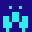# FIXED

Fixed point number package

Dr. Heinrich Hohl <hohl@isartext.de>

Version 1.0.0 - 2016-10-01

## Introduction

This package provides words for convenient handling of fixed point numbers.

A fixed point number (f) is an integer number with an implied decimal point at a specified position. Fixed point numbers may be used instead of floating point numbers if only basic arithmetic operations (+, -, *, /) are required in an application.

What does implied decimal point mean?

Let us assume that your application deals with weight (in kg) and price (in EUR). In this case you would store weights and prices as follows:

• Weight: 1 kg = 1.000 kg = 1000 g = 1000
• Price: 1 EUR = 1.00 EUR = 100 ct = 100

Although weight and price are officially shown in kg and EUR, they are internally stored in grams and cents. Before you input or output a fixed point number, you must specify the position of the implied decimal point. In the above example these positions are 3 and 2 digits from the right, respectively.

## Versions

The package is available in versions for the following FORTH systems:

System Standard Type Files
PC/FORTH (LMI) Forth-83 16-bit fixed_lmi.txt
SwiftForth (Forth, Inc.) ANS Forth 32-bit fixed_sf.f
VFX Forth (MPE) ANS Forth 32-bit fixed_vfx.fth

The package was developed under LMI PC/FORTH in 1992 and published in the German Forth magazine Vierte Dimension Nr. 2/1992, p.7. The LMI version is included for completeness and because someone might still want to use the package on a 16-bit system.

It is also instructive to see how much simpler the code became after rewriting the package for 32-bit systems. The following description will concentrate on the 32-bit versions (SwiftForth, VFX Forth) of the package.

## Installation

Use the following commands to install the package:

• SwiftForth: `INCLUDE fixed_sf.f`
• VFX Forth: `INCLUDE fixed_vfx.fth`

This makes the fixed point words available. After loading you still have a standard system. The number conversion routine of the Forth system is not modified by this package.

## Glossary

The package adds the following words to the system:

``PLACES  FIXED  (F.)  F.  F.R``

See glossary.md for stack comments and descriptions of the defined words.

## Usage

Use `PLACES` to specify the number of places behind the decimal point. Input a number (with or without decimal point), immediately followed by `FIXED` which converts the input to a fixed point number. It does this by appending zeros or by truncating excessive digits behind the decimal point as required. The number is now in a well defined format and can be stored, used for calculations, or output.

### Examples

``````3 PLACES

6 FIXED  F.                   --> 6.000 ok
2.4938 FIXED  F.              --> 2.493 ok

6 FIXED  2.4938 FIXED  +  F.  --> 8.493 ok

8.493  1 PLACES  F.           --> 8.4 ok

: .WEIGHT ( f -- )  3 PLACES F. ;
: .PRICE  ( f -- )  2 PLACES F. ;``````

### Important rules

1. Do not put any other words between the number input routine and `FIXED`. Depending on the FORTH system, words such as `.S` may alter the contents of `DPL`.

2. On 32-bit systems, fixed point numbers are represented by single length numbers.

3. Use standard words for storage (`@ ! CONSTANT VALUE VARIABLE`) and for stack manipulation (`DUP DROP SWAP OVER ROT` etc.)

4. Use `+` and `-` to add and subtract two fixed point numbers, respectively. Both operands must be based on the same number of places, and the result will inherit this number of places.

5. Use `*` and `/` to multiply or divide two fixed point numbers. The operands and the result are generally based on different numbers of places. Afterward, you must scale the result by a suitable power of ten.

## Conformance

This package largely conforms to Forth-83 (LMI) and ANS Forth (SwiftForth, VFX). Nonstandard words that should be mentioned:

• `DPL` contains the decimal point location after an integer number conversion has been performed
• `{ ... }` marks block comments in SwiftForth
• `(* ... *)` marks block comments in VFX Forth
• `PACKAGE PRIVATE PUBLIC END-PACKAGE` are used for information hiding in SwiftForth
• `MODULE EXPORT END-MODULE` are used for information hiding in VFX Forth

## by HeinrichHohl